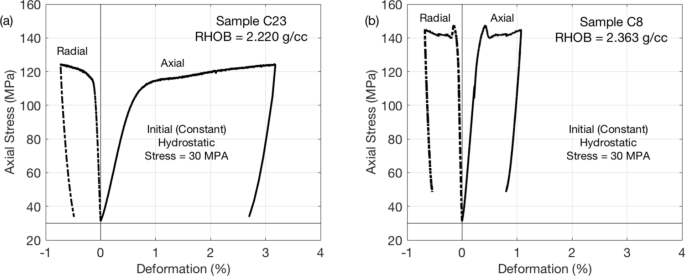# Effect of heterogeneity on failure of natural rock samples[ad_1]

The predominant mode of the failure of natural rock is fracturing. The amount of past work on this topic is massive. Classic papers and monographs1,2,3,4 have been followed by a plethora of recent publications describing experimental results, both physical and computational. Experiments on artificial layered rock samples5 indicate that failure cracks often appear along layer interfaces. Similar results are reported for slate samples6.

A “single plane of weakness” rock failure theory7 based on earlier observations8 refers to an isotropic and homogeneous material cut through by a plane with reduced shear strength. Failure under axial load occurs on this plane. Later papers, such as9, invoke this theory as well.

By and large, the abovementioned references, together with other numerous publications, experimental and theoretical, link rock failure to the presence of meso-scale anisotropy and inhomogeneity in rock samples.

In contrast, for an isotropic and homogeneous rock, classic strength of materials theory gives the following expression for the shear stress (tau) along the plane inclined at angle (theta) to the horizontal under uniaxial loading where the principal vertical stress (sigma_{3}) exceeds the two equal horizontal stresses (sigma_{1}) = (sigma_{2}):

$$tau = frac{{sigma_{3} – sigma_{1} }}{2}sin 2theta ,$$

(1)

a formula conveniently visualized by the Mohr circle (Fig. 1).Figure 1

According to Eq. (1), the maximum shear stress occurs on the plane cutting the sample at 45° to the horizontal. However, a carbonate sample under examination subjected to uniaxial loading developed a subhorizontal crack inclined at about 20° (Fig. 1c). What could have caused such a counterintuitive behavior?

### Tests and results

Carbonate samples C23 and C8 extracted from a well drilled in a Saudi oil field were cleaned and dried in the laboratory. Their dynamic Poisson’s ratio (nu) and Young’s modulus (E) were computed from the bulk density (rho_{b}) and the P- and S-wave velocities, (V_{p}) and (V_{s}), measured at 30 MPa hydrostatic stress as

$$nu = frac{1}{2}frac{{(V_{p} /V_{s} )^{2} – 2}}{{(V_{p} /V_{s} )^{2} – 1}};quad E = 2rho_{b} V_{s}^{2} (1 + nu )$$

(2)

and are listed, together with the depth of their original location, in Table 1.

Both samples were first hydrostatically loaded to 30 MPa. Afterwards, the vertical stress was gradually increased until failure, with the confining stress remaining constant. Finally, after a period of post-failure deformation, the samples were axially unloaded to the initial hydrostatic stress 30 MPa. All tests were performed using a load-frame Autolab-1500 supplied by New England Research. The elastic-wave velocities were measured on room-dry samples using ultrasonic transducers with 750 kHz central frequency. The axial and radial deformations were measured by linear variable differential transformers (LVDT). The results of these tests are shown in Fig. 2.Figure 2

In both tests, observed was a textbook behavior with almost linearly elastic deformation followed by failure, plastic deformation, and elastic unloading. The unloading curves essentially parallel the loading curves. The post-yield flow time for C23 was 30 min, while it was 8 min for C8. This explains why the post-yield deformation in C23 was about three times that in C8.

### First sample: post-test and pre-test images

Post-test CT-scan images (vertical slices of the original 3D images taken along the central axis) for sample C23 are shown in Fig. 3. The major crack in the lower half of the sample is inclined at about 20° to the horizontal and surrounded by minor cracks at the circumference of the sample.Figure 3

The pre-test image (also a vertical slice of the original 3D image taken along the central axis) shown in the same figure, exhibits a clear CT-value contrast at the position where the crack later developed. These CT values indicate a density and, hence, porosity contrast and, as a result, the elastic moduli contrast with the stiffer part of the sample located above its softer part.

[ad_2]

Source link